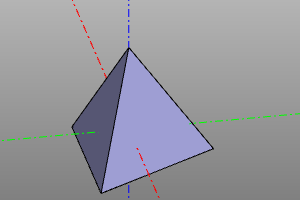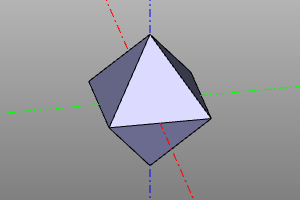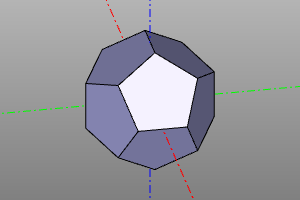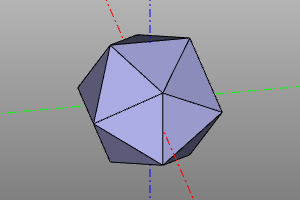# Volumetric bodies

This section provides basic CSG geometry primitives.

## Box

The volumetric body is a parallelepiped. It is set with the indication of three sizes x, y, z. Specifying one dimension a generates a cube (a, a, a). Setting the boolean option center aligns the geometric center of the body with the origin.

Сигнатуры:

``````box(x, y, z, center=True/False)
box(size=(x,y,z), center=True/False)
box(size=a, center=True/False)
``````

Примеры:

``````box(10, 20, 30, center=False)
box(size=(10,20,30), center=False) # alternate
box(10, center=True)
``````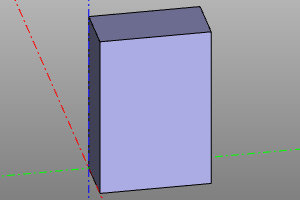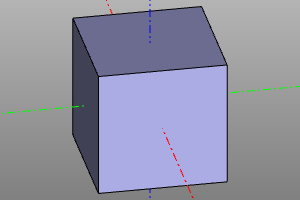## Sphere

The volumetric body is a sphere. Specified by specifying the radius. It is possible to construct a sector of a sphere using the optional parameters yaw, pitch.

Signature:

``````sphere(r=radius, yaw=yaw, pitch=(minPitch, maxPitch))
``````

Примеры:

``````sphere(10)
sphere(10, yaw=math.pi*2/3)
sphere(10, pitch=(deg(20), deg(60)))
sphere(10, yaw=deg(120), pitch=(deg(20), deg(60)))
``````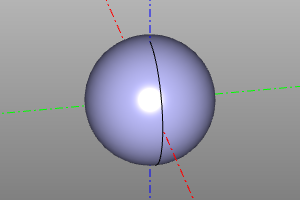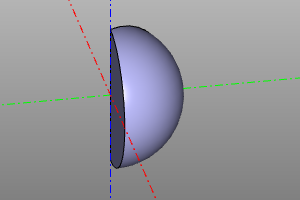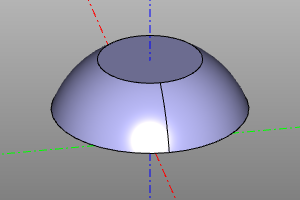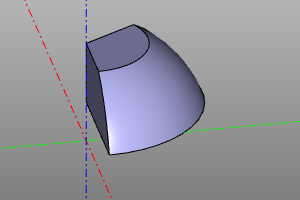## Cylinder

The volumetric body is a cylinder. It is set with the indication of the radius and height. It is possible to build a cylinder sector using the optional yaw parameter. Setting the center option aligns the geometric center of the body with the origin.

Signature:

``````cylinder(r=radius, h=height, yaw=yaw, center=True/False)
``````
``````cylinder(r=10, h=20)
cylinder(r=10, h=20, yaw=deg(45))
cylinder(r=10, h=20, center=True)
cylinder(r=10, h=20, yaw=deg(45), center=True)
``````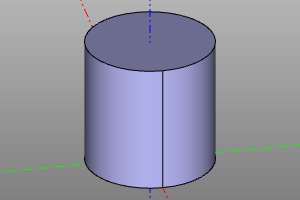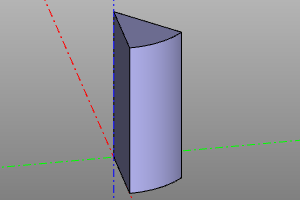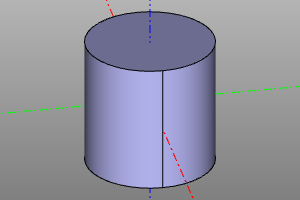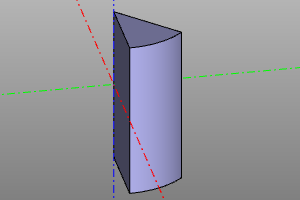## Cone

The volumetric body is a cone. It is specified by specifying the lower radius r1, upper radius r2 and height. It is possible to create a cone sector using the optional yaw parameter. Setting the center option aligns the geometric center of the body with the origin. The radii r1 and r2 can be zero, which corresponds to a pointed cone.

Signature:

``````cone(r1=botRadius, r2=topRadius, h=height, yaw=yaw, center=True/False)
``````

Примеры:

``````cone(r1=20, r2=10, h=20)
cone(r1=20, r2=10, h=20, yaw=deg(45))
cone(r1=0, r2=20, h=20)
cone(r1=20, r2=0, h=20, center=True)
``````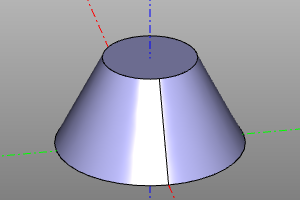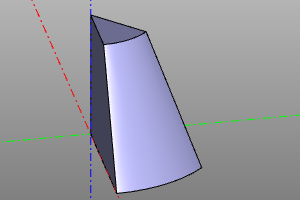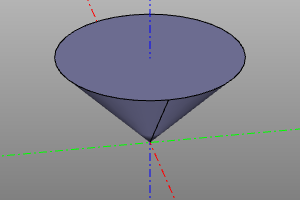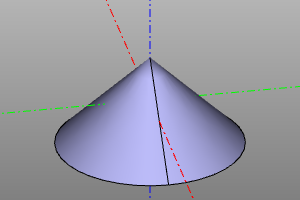## Torus

The volumetric body is a torus. It is specified by specifying the central radius r1 and the local radius r2. It is possible to construct torus sectors using the optional parameters yaw, pitch.

If the interval of the pitch angle does not contain an inner region, a corresponding cylindrical insert is formed in the center. If the interval of the pitch angle does not contain an outer region, the corresponding part of the torus is bounded by the plane.

Signature:

``````torus(r1=centralRadius, r2=localRadius, yaw=yaw, pitch=(minPitch, maxPitch))
``````

Примеры:

``````torus(r1=20, r2=5)
torus(r1=20, r2=5, yaw=deg(120))
torus(r1=20, r2=5, pitch=(deg(-20), deg(120)))
torus(r1=20, r2=5, pitch=(deg(-20), deg(120)), yaw=deg(120))
torus(r1=20, r2=5, pitch=(deg(-140), deg(140)), yaw=deg(120))
torus(r1=20, r2=5, pitch=(deg(-20), deg(190)), yaw=deg(120))
``````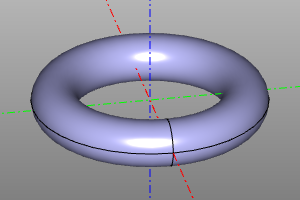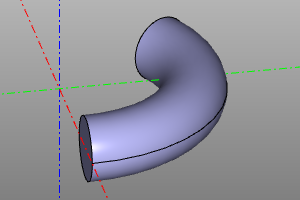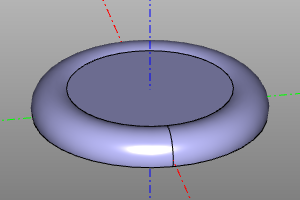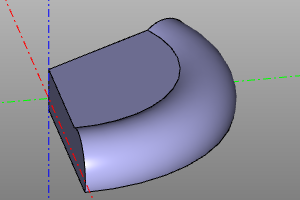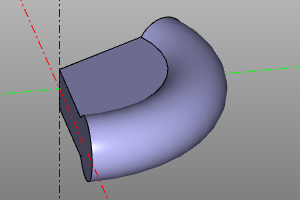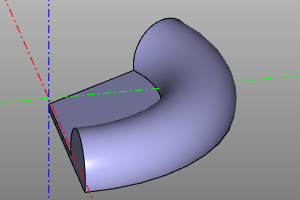A special volumetric body, which is the lower half-space. Like other solid bodies, it supports transformations and, using them, can represent any possible half-space. Unlike ordinary bodies, it cannot be displayed directly. Used in conjunction with the difference and intersection operations.

``````sphere(r=10) - halfspace().rotateX(deg(150))
sphere(r=10) ^ halfspace().rotateX(deg(150))
``````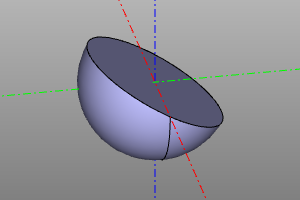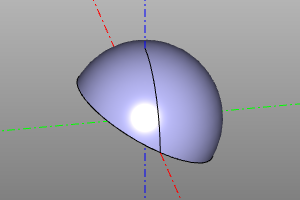## Platonic solids

Construction of Platonic solids. The library is based on https://github.com/qalle2/plato.scad

Regular polyhedron Number of vertices Number of edges Number of faces Number of sides at a face Number of edges adjacent to a vertex Type of spatial symmetry
Tetrahedron 4 6 4 3 3 Td
Hexahedron 8 12 6 4 3 Oh
Octahedron 6 12 8 3 4 Oh
Dodecahedron 20 30 12 5 3 Ih
Icosahedron 12 30 20 3 5 Ih

The library allows you to specify the dimensions of bodies through the radius of the circumscribed circle r or through the length of the edge a.

Сигнатуры:

``````zencad.tetrahedron(r=1, a=None, shell=False)

# Альтернативный синтаксис
``````

Example:

``````# Через радиус:
tetrahedron(10)
hexahedron(10)
octahedron(r=10)
dodecahedron(r=10)
icosahedron(10)

# Через длину ребра:
icosahedron(a=10)

# Альтернативный синтакис: